Linear Ordinary Differential Equations of First and Second Order - 3

# Linear Ordinary Differential Equations of First and Second Order - 3 - Notes | Study Physics for IIT JAM, UGC - NET, CSIR NET - Physics

 1 Crore+ students have signed up on EduRev. Have you?

Exact Equations

Review on partial derivatives and Chain Rules for functions of 2 variables.

Consider a function f (x, y). We use these notations for the partial derivatives: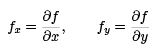and correspondingly the higher derivatives: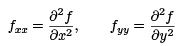and the cross derivatives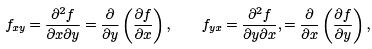where we have the identity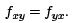Now, consider x = x(t) and y = y(t), and we form a composite function as f (x(t), y(t)).

We see that f now depends only on t.

Chain Rule: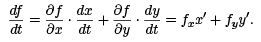Example 1. Let f (x, y) = x2y2 + ex, and x(t) = t2, y(t) = et , and consider the composite function f (x(t), y(t)). Compute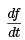Answer. We ﬁrst compute the derivatives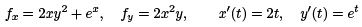By the Chain Rule, we compute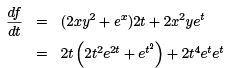Special case: If y = y(x), then the composite function f (x, y(x)) will follow this form of Chain Rule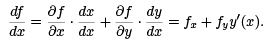Example 2. Let y(x) be the unknown. Consider the equation

6x + exy2 + 2exyy′ = 0

We see that the equation is NOT linear. It is NOT separable either. None of the methods we know can solve it.

However, deﬁne the function

ψ(x, y) = 3x2 + exy2

We notice that

ψx = 6x + exy2, ψy = 2ex y and the equation can be written as

ψx (x, y) + ψy (x, y)y′ = 0.

Since y = y(x), we apply the Chain Rule to the composite function ψ(x, y(x)) and get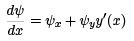which is the left-hand side of the equation. By the diﬀerential equation, we now have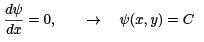where C is an arbitrary constant, to be determined by initial condition. We have found the solution in an implicit form :

3x2 + exy= C.

In this example, we are even able to write out the solution in an explicit form by algebraic manipulation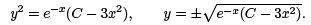Here, the choice of + or  − sign should be determined by initial condition.

Deﬁnition of an exact equation. En equation in the form

M (x, y) + N (x, y)y′ = 0

is called exact if there exists a function ψ(x, y) such that ψx = M and ψy = N .

How to check if an equation is exact? By the identity ψxy = ψyx, we must have My (x, y) = Nx(x, y).

How to solve it? Need to ﬁnd the function ψ, then we get implicit solution

ψ(x, y) = C.

How to ﬁnd ψ? By using the facts that

ψx = M (x, y), ψy = N (x, y) and integrate. We will see this through an example.

Example 3. Check if the following equation is exact (2x + 3y) + (x − 2y)y′ = 0.

M (x, y) = 2x + 3y, N (x, y) = x − 2y.

Then

My = 3, Nx = 1, M≠ Nx,

so the equation is not exact.

Remark 1. Consider a separable equation: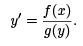Multiply both sides by g(y) and re-arranging terms, we get

f (x) − g(y)y′ = 0.

Now we check if this equation is exact. Clearly, we have fy = 0 and gx = 0, so it is exact. We may conclude that all separable equations can be rewritten into an exact equation.

Remark 2. However, the exactness of an equation is up to manipulation. Consider the separable equation in Remark 1, and rewrite the equation now into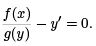So now we have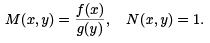Since Nx = 0 and My = 0 in general, the equation is not exact.

So, be careful. When you say an equation is exact, you must specify in which form you present your equation.

Example 4. Consider the equation

(2x + y) + (x + 2y)y′ = 0

(1) Is it exact? (2) If yes, ﬁnd the solution with the initial condition y(1) = 1.

Answer.(1).  We have M = 2x + y and N = x + 2y, so My = 1 and Nx = 1, so the equation is exact.

(2). To solve it, we need to ﬁnd the function ψ.

We have ψx = 2x + y, ψy = x + 2y.             (A)

Integrating the ﬁrst equation in x: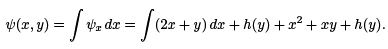(Review: To perform a partial integration in x, we treat y as a constant. Therefore, the integration constant could depend on y since it is a constant. That’s why we add h(y), a function of y, to the anti-derivative.)

To determine h(y), we use the second equation in (A).

ψy = x + h′ (y) = x + 2y, h′ (y) = 2y, h(y) = y2.

Therefore

ψ = x2 + xy + y2

and the implicit solution is

x2 + xy + y2 = C.

Finally, we determine the constant C by initial condition. Plug in x = 1, y = 1, we get C = 3, so the implicit solution is

x2 + xy + y2 = 3.

Example 5. Given equation

(xy2 + bx2 y) + (x + y)x2 y′ = 0. (1)

Find the values of b such that the equation is exact. (2) Solve it with that value of b.

M (x, y) = xy2 + bx2y, N (x, y) = x3 + x2y

so

My = 2xy + bx2, Nx = 3x+ 2xy

We see that we must have b = 3 to ensure My = Nx which would make the equation exact. (2). We now set b = 3. To solve the equation, we need to ﬁnd the function ψ. We have

ψ= xy2 + 3x2y, ψy = x+ x2y.

Integrating the ﬁrst equation in x: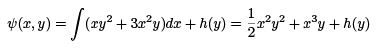To ﬁnd h(y), we use ψy :

ψy = x2y + x3 + h′(y) = N= 3x+ 2xy

We must have h′ (y) = 0, so we can use h(y) = 0.

The implicit solution is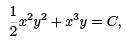where C is arbitrary, to be determined by initial condition.

The document Linear Ordinary Differential Equations of First and Second Order - 3 - Notes | Study Physics for IIT JAM, UGC - NET, CSIR NET - Physics is a part of the Physics Course Physics for IIT JAM, UGC - NET, CSIR NET.
All you need of Physics at this link: Physics

## Physics for IIT JAM, UGC - NET, CSIR NET

159 docs
 Use Code STAYHOME200 and get INR 200 additional OFF

## Physics for IIT JAM, UGC - NET, CSIR NET

159 docs

Track your progress, build streaks, highlight & save important lessons and more!

,

,

,

,

,

,

,

,

,

,

,

,

,

,

,

,

,

,

,

,

,

,

,

,

,

,

,

;